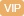| APP | 校园号 | 客服 客服热线：400-863-9889

400-863-9889（2015秋•广州期中）计算下面各题．（能简算的要简算）
0.98×100.1
146.5-（23+46.5）
10÷0.2÷0.5
64×4.5+36×4.5
0.125×32×2.5
【考点】运算定律与简便运算小数四则混合运算【专题】运算顺序及法则；运算定律及简算．

（7首先计算除法然计算法，求出算式的值是即可．
【分析】根据乘法交换律和结简即可．
（）首先计算小括号里面，然后计算小括外面，求出式的值少即可．
【解答】解：02×3.86×4
=98
16.7×21+1.8
=4587
=9.×10
9.85.69.8×44
=.43×10+0.3×2
=386
=4.86
30×3
9.45-0450.1
（）.7÷（1.5×0.8）
=61
=430.86
=7.1×10
=0.43×（00+）
=.8×5.6+44）
=9.45
3.7÷1
.117-76.1×7
76.1×（17-）
=.7
【点评】此题主考查小数四则合运算，注意运算顺，注意乘运算定的应．

0/0